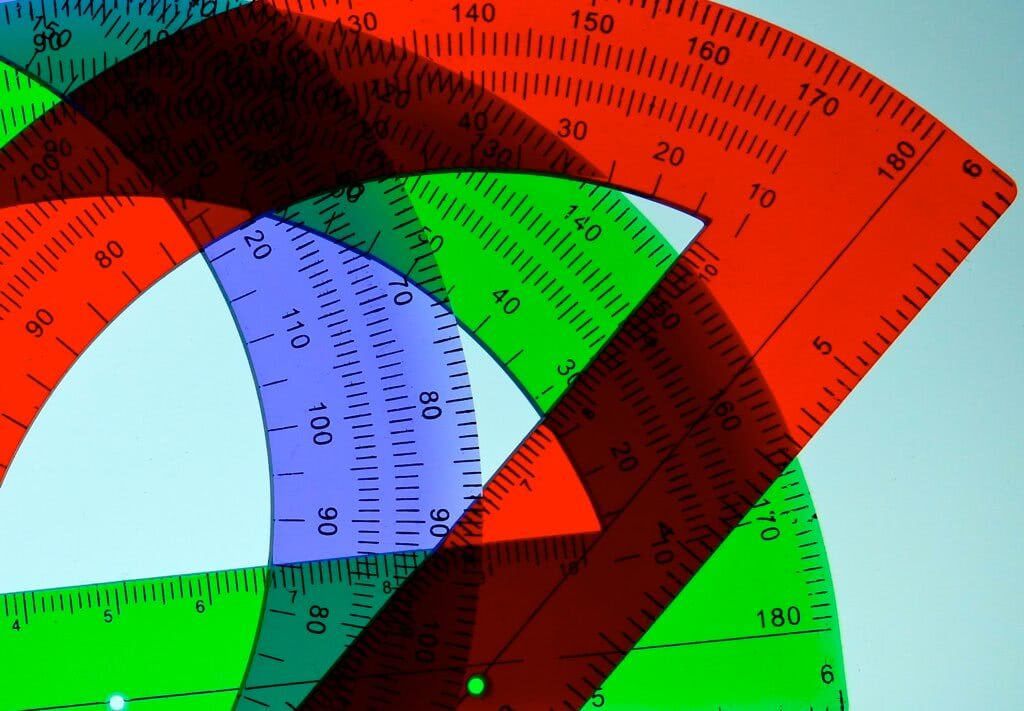# New SAT Math Test Prep: Here’s What You Need to KnowSource: Flickr user deanhochman.

The new SAT math section has been entirely revamped. There are now 54 questions to be answered in 70 minutes. Much like the language section of the SAT, the math test now requires test takers to utilize problem solving skills with real world applications. This means that there might be multiple steps required in order to calculate the correct answer. After all, you cannot remodel your kitchen after having only calculated the length of one wall.

## What’s In the New SAT Math Test

The new math test consists of two different sections: Math Test – Calculator and Math Test – No Calculator. Note, there will be questions on the calculator portion that are geared towards measuring your ability to reason. These questions can be answered more efficiently when not using the calculator.

In addition to the two test sections, there are also two types of answers. Most of the questions will still have multiple choice answers. Those that do not, will now have what are called “grid-ins”. Questions with grid-in answers require the test taker to come up with their own answer instead of selecting the answer from a list. After the problem has been solved, and an answer found, it is written in the boxes (grid) and the corresponding bubbles filled in below. For more information on this, see College Board’s example (toward the bottom of the page).

The new SAT Math test will also include problems where information is given (e.g., table, chart, diagram) and multiple questions are asked regarding the same figure or scenario. These questions will be a similar format to those in the Reading/Writing sections of the SAT.

## What You Need to Know for the New SAT Math Test

College Board has broken the major, must-know math concepts down into 3 areas. They have named them the Heart of Algebra, Problem Solving and Data Analysis, and Passport to Advanced Math. Not included in these categories, but still found on the test, are geometry and trigonometry as it is still related to college coursework and many careers.

Both the calculator and no-calculator tests will have questions from all three categories. Furthermore, each category will contain questions with multiple choice and grid-in answers.

1. Heart of Algebra. Questions that fall into this category are those assessing core algebra concepts. Systems of equations, linear equations, and inequalities are the three main components that make up this section. Also included in this portion is graphing, and how changes in an equation will affect the shape of the graph.
2. Problem Solving & Data Analysis. These are the questions that you will likely encounter graphs, charts, and other figures in. According to College Board’s description, these problems “are likely to draw from science and social science” contexts. Concepts included in this section are: ratios, percentages, unit conversion, correlations, linear and exponential growth, probability, and statistics.
3. Passport to Advanced Math. As the name implies, the concepts in this category are those associated with more advanced math. The equations become more complex, as do the functions the test taker will be asked to create and manipulate. The questions in this section cover topics such as quadratic equations, polynomials (factoring, adding/subtracting, multiplying/dividing), nonlinear relationships between variables, and more.

Two tests, two answer types, and three sub-sections make up the new SAT math test score. Again, this test will be administered for the first time on March 5, 2016. Class of 2016, you are the last group to take the current SAT. Classes 2017 and beyond, you will be taking the new version.

For those in the process of studying, College Board has provided an introduction to the new math test, including directions for grid-in answers, and sample questions. I also discovered a perfect scorer’s youtube account where practice math tests are broken down, and answers are explained.

If you are in need of a more comprehensive SAT test preparation interface, check out our list of SAT test prep resources.

Good luck!